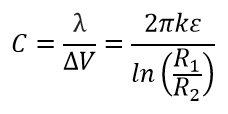# Capacitance of Cylindrical Capacitor

For a cylindrical geometry the charge resides on the outer surface of the inner conductor and the inner surface of the outer conductor. The capacitance for cylindrical conductors can be calculated by evaluating the voltage difference between the conductors for a given charge on each of them.

m
m

#### Result

F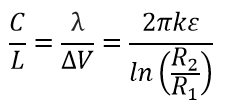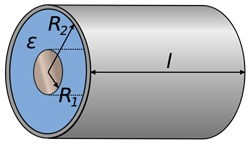Where,
ε=εo εr
εo=8.854187817 x 10-12
εr= Relative Permittivity

##### Formula Derivation:
The capacitance for cylindrical conductors can be calculated by evaluating the voltage difference between the two conductors for a given charge on each. We use Gauss' law and assume the cylinders to have an infinite length in a vacuum. The electric field outside a charged cylinder can be calculated by: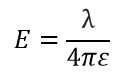The voltage between the cylinders can be found by integrating the electric field along the radius: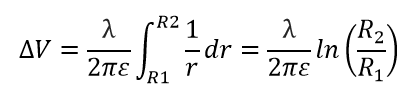We can calculate the capacitance per unit length to be: